# Themes, topics - math word problems

1. The painterIn order for the painter to get the desired color, he must mix green and yellow in a ratio of 4: 7. If it has 28 l of green color, how many liters of yellow color should he add? How many liters of mixed color does he get?
2. SoldierAn experienced soldier digs a good trench in 30 hours. Two soldiers dug the same trench each 40 hours. How many hours will the ditch dig all three together?
3. The HotelThe Holiday Hotel has the same number of rooms on each floor. Rooms are numbered with natural numbers sequentially from the first floor, no number is omitted, and each room has a different number. Three tourists arrived at the hotel. The first one was in r
4. WorkersTwo workers will construct the stage at the festival in 54 hours. Organizers need to shorten the assembly time by 42 hours. How many workers must the assembly company send?
5. Tractors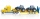5 tractors will plow the field for 8 hours. In 2 hours, 1 tractor broke down. How long do the remaining tractors complete the plow?
6. CuSO4 mixtureHow many grams of solid CuSO4 we have to add to 450g of 15% CuSO4 solution to produce a 25% solution?
7. Car driver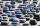The car driver is in town A and is scheduled to be in town B at a specified hour. If it travels at an average speed of 50km/h, it will arrive in city B 30 minutes later. But if he travels at an average speed of 70km/h, he would arrive half an hour earlier
8. Construct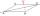Construct a rhombus ABCD, if the size of the diagonal AC is 6 cm and diagonal BD 8 cm long.
9. The train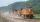The train passed the observer in the 20s and passed through a 1500m tunnel in 2 minutes. Calculate the speed (km/h) and train length.
10. Three paintersOne painter would have painted the fence for 15 hours, the second in 12 hours. The plot had to be painted in four hours, so they called the third one, and all worked together. For what time would the third painter paint fence the fence alone?
11. Two pipesHow long will the pool be filled with a double supply pipe if it takes the pool to fill the first pipe by 4 hours longer and the second pipe 9 hours longer than both pipes open at the same time?
12. PeroxideHow many ml 30% of peroxide (H2O2) should be poured into 100ml H2O to give a 20% solution?
13. Two pumps togetherThe first pump will fill the tank itself in 3 hours and the second one in 6 hours. How many hours will the tank be full if both pumps are worked at the same time?
14. Orlík hydroelectric plantThe Orlík hydroelectric power plant, built in 1954-1961, consists of four Kaplan turbines. For each of them, the water with a flow rate of Q = 150 m3/s is supplied with a flow rate of h = 70.5 m at full power. a) What is the total installed power of the p
15. Together 3If Sally can paint a house in 4 hours, and John can paint the same house in 6 hours, how long will it take for both of them to paint the house together?
16. Our houseOur house would paint four painters in 14 days. How long could it paint five painters? How long will it take if two other painters will come to help after 5 days?
17. Train and walker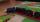Walker passes an hour 5 km. Express train per hour 100 km. Walker went 4 hours. How many minutes took the distance express train?
18. Cyclist 9A cyclist travels at a a speed of 4.25 km per hour. At that rate, how far can he travel in 3.75 hours?
19. Electric input powerSolve problems related to electric power: a) U = 120 V, I = 0.5 A, P =? b) P = 200 W, U = 230 V, I =? c) I = 5 A, P = 2200W, U =?
20. Two diggersTwo diggers should dig a ditch. If each of them worked just one-third of the time that the other digger needs, they'd dig up a 13/18 ditch together. Find the ratio of the performance of this two diggers.

Do you have an interesting mathematical word problem that you can't solve it? Enter it, and we can try to solve it.

To this e-mail address, we will reply solution; solved examples are also published here. Please enter the e-mail correctly and check whether you don't have a full mailbox.

Please do not submit problems from current active competitions such as Mathematical Olympiad, correspondence seminars etc...